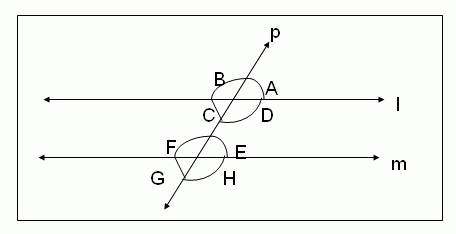Email us to get an instant 20% discount on highly effective K-12 Math & English kwizNET Programs!

#### Online Quiz (WorksheetABCD)

Questions Per Quiz = 2 4 6 8 10

### Middle/High School Algebra, Geometry, and Statistics (AGS)5.9 Parallel Lines - Corresponding and Alternative Angles

 Axiom - 2: Given a line and a point not on it, there exists one and only one line parallel to given line through the given point. This means that if 'l' is a given line and 'P' is a given point not on 'l', we can draw through 'P' one and only one line parallel to 'l'. Note: I. Corresponding Angles: Two angles are said to be pair of corresponding angles if: i. They are on the same side of the transversal. ii. One is an interior angle and the other is an exterior angle. iii. They are not adjacent angles. II. Alternate Angles: Two angles are said to be a pair of alternate angles if: i. both are interior angles. ii. They are on either side of the transversal. iii. They are not adjacent angles. Axiom - 3:[Axiom of Corresponding Angles] If a transversal intersects two coplanar parallel lines, then the corresponding angles are equal. This means that if 'l' and 'm' are two parallel lines and a transversal 'p' intersects them. Then the following pairs of corresponding angles are equal. 1. Đ A = Đ E 2. Đ D = Đ H 3. Đ B = Đ F 4. Đ C = Đ GTheorem - II: If a transversal intersects two parallel lines, then the alternative angles are equal.Hypothesis: Two parallel lines 'l' and 'm' are intersected by a transversal 'p'. ĐC, ĐE and ĐD, ĐF are the two pairs of alternative angles formed. Conclusion: a. ĐC = ĐE. b. Đ D = ĐF. Proof: We know that ĐC and ĐB form a linear pair (Pair of angles formed on a straight line) and ĐE and ĐF also formed a linear pair. Therefore, ĐC + ĐB = 180° and ĐE + ĐF = 180° Therefore, ĐC + Đ B = ĐE + ĐF But Đ B = Đ F (by the axiom of corresponding angles). Therefore, ĐC = ĐE [By cancelling equal angles ĐB and ĐF both sides]. Therefore, similarly we can be shown that ĐD = ĐF. Directions: Draw parallel lines and a transversal and show corresponding angles and alternate angles.